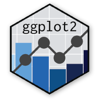Show Sidebar Hide Sidebar# geom_count in ggplot2

How to make a 2-dimensional frequency graph in ggplot2 using geom_count Examples of coloured and facetted graphs.

### New to Plotly?

Plotly's R library is free and open source!
You can set up Plotly to work in online or offline mode.
We also have a quick-reference cheatsheet (new!) to help you get started!

### Version Check

Version 4 of Plotly's R package is now available!
Check out this post for more information on breaking changes and new features available in this version.

library(plotly)
packageVersion('plotly')

##  '4.9.1'


### Basic geom_count Plot

geom_count is a way to plot two variables that are not continuous. Here's a modified version of the nycflights13 dataset that comes with R; it shows 2013 domestic flights leaving New York's three airports. This graph maps two categorical variables: which of America's major airports it was headed to, and which major carrier was operating it.

It's good to show the full airport names for destinations, rather than just the airport codes. You can use aes(group = ), which doesn't modify the graph in any way but adds information to the labels.

library(plotly)
flightdata <- read.csv("https://raw.githubusercontent.com/plotly/datasets/master/flightdata.csv", stringsAsFactors = FALSE)

p <- ggplot(flightdata, aes(y=airline, x=dest, colour = dest, group=airport)) +
geom_count(alpha=0.5) +
labs(title = "Flights from New York to major domestic destinations",
x = "Origin and destination",
y = "Airline",
size = "")

ggplotly(p)


By using facets, we can add a third variable: which of New York's three airports it departed from. We can also colour-code by this variable.

library(plotly)
flightdata <- read.csv("https://raw.githubusercontent.com/plotly/datasets/master/flightdata.csv", stringsAsFactors = FALSE)

p <- ggplot(flightdata, aes(y=airline, x=origin, colour=origin, group=airport)) +
geom_count(alpha=0.5) +
facet_grid(. ~ dest) +
labs(title = "Flights from New York to major domestic destinations",
x = "Origin and destination",
y = "Airline",
size = "")

ggplotly(p)


### Customized appearance

The airport labels at the bottom aren't very visible and aren't very important, since there's a colour key to the side; we can get rid of the text and ticks using theme() options. Let's also use the LaCroixColoR package to give this geom_count chart a new colour scheme.

library(plotly)
library(LaCroixColoR)
flightdata <- read.csv("https://raw.githubusercontent.com/plotly/datasets/master/flightdata.csv", stringsAsFactors = FALSE)

p <- ggplot(flightdata, aes(y=airline, x=origin, colour=origin, group=airport)) +
geom_count(alpha=0.5) +
facet_grid(. ~ dest) +
scale_colour_manual(values = lacroix_palette("PassionFruit", n=3)) +
theme(axis.text.x = element_blank(),
axis.ticks.x = element_blank()) +
labs(title = "Flights from New York to major domestic destinations",
x = "Origin and destination",
y = "Airline",
size = "")

ggplotly(p)


### geom_count vs geom_point

Here's a comparison of geom_count and geom_point on the same dataset (rounded for geom_count). Geom_point has the advantage of allowing multiple colours on the same graph, as well as a label for each point. But even with a low alpha, there are too many overlapping points to understand what the actual distribution looks like, only a general impression.

library(plotly)
library(dplyr)
beers <- read.csv("https://raw.githubusercontent.com/plotly/datasets/master/beers.csv", stringsAsFactors = FALSE)

df <- beers %>%
mutate(abv = round(abv*100),
ibu = round(ibu/10)*10) %>%
filter(!is.na(style2))

p <- ggplot(df, aes(x=abv, y=ibu, colour=style2)) +
geom_count(alpha=0.5) +
theme(legend.position = "none") +
facet_wrap(~style2)

ggplotly(p)

library(plotly)
library(dplyr)
beers <- read.csv("https://raw.githubusercontent.com/plotly/datasets/master/beers.csv", stringsAsFactors = FALSE)

df <- filter(beers, !is.na(style2))

p <- ggplot(df, aes(x=abv, y=ibu, colour=style2)) +
geom_point(alpha=0.2, aes(text = label)) +
theme(legend.position = "none") +
facet_wrap(~style2) +
labs(y = "bitterness (IBU)",
x = "alcohol volume (ABV)",
title = "Craft beers from American breweries")

ggplotly(p)